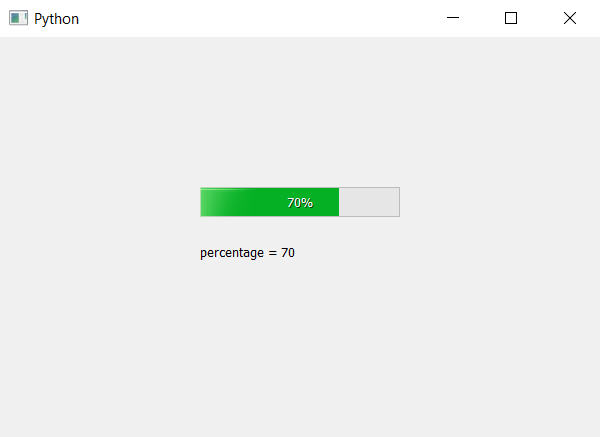# PyQt5 – How to get percentage of Progress Bar ?

In this article we will see how to get the percentage value of progress bar, we can set the percentage of progress bar using `setValue` method. In order to get the percentage value we use `text` method which will return the integer that indicates the percentage.

Syntax : bar.text()

Argument : It takes no argument.

Return : It return integer.

Below is the implementation.

 `# importing libraries ` `from` `PyQt5.QtWidgets ``import` `*`  `from` `PyQt5 ``import` `QtCore, QtGui ` `from` `PyQt5.QtGui ``import` `*`  `from` `PyQt5.QtCore ``import` `*`  `import` `sys ` ` `  ` `  `class` `Window(QMainWindow): ` ` `  ` `  `    ``def` `__init__(``self``): ` `        ``super``().__init__() ` ` `  `        ``# setting title ` `        ``self``.setWindowTitle(``"Python "``) ` ` `  `        ``# setting geometry ` `        ``self``.setGeometry(``100``, ``100``, ``600``, ``400``) ` ` `  `        ``# calling method ` `        ``self``.UiComponents() ` ` `  `        ``# showing all the widgets ` `        ``self``.show() ` ` `  `    ``# method for widgets ` `    ``def` `UiComponents(``self``): ` ` `  `        ``# creating progress bar ` `        ``bar ``=` `QProgressBar(``self``) ` ` `  `        ``# setting geometry to progress bar ` `        ``bar.setGeometry(``200``, ``150``, ``200``, ``30``) ` ` `  `        ``# set value to progress bar ` `        ``bar.setValue(``70``) ` ` `  `        ``# getting percentage value ` `        ``p_value ``=` `bar.text() ` ` `  `        ``# creating label to print percentage ` `        ``label ``=` `QLabel(``"percentage = "` `+` `str``(p_value), ``self``) ` ` `  `        ``# moving the label ` `        ``label.move(``200``, ``200``) ` ` `  `        ``# setting alignment to centre ` `        ``bar.setAlignment(Qt.AlignCenter) ` ` `  ` `  `# create pyqt5 app ` `App ``=` `QApplication(sys.argv) ` ` `  `# create the instance of our Window ` `window ``=` `Window() ` ` `  `# start the app ` `sys.exit(App.``exec``()) `

Output :Whether you're preparing for your first job interview or aiming to upskill in this ever-evolving tech landscape, GeeksforGeeks Courses are your key to success. We provide top-quality content at affordable prices, all geared towards accelerating your growth in a time-bound manner. Join the millions we've already empowered, and we're here to do the same for you. Don't miss out - check it out now!